# Table ⚠️ Brady: Values of sinus, cosine, tangent, Kotangens

## What is Bradys table

The use of calculators with complex calculations (for example, formulas with logarithms) today is considered the default standard. But another 20-30 years ago, when computing equipment was disseminated not so much, other calculation methods came to the rescue - with the help of special tables, a logarithmic line or an arithmometer.

Definition

Table Brady - A mathematical manual in which the tables needed for work on the course of mathematics and for practical computing created by Vladimir Modestovic Brady.

They received their name from the "Four-digit Mathematical Tables" brochure compiled by Vladimir Braradis. The book was repeatedly reprinted in Soviet times with large circulations (up to 500,000 copies) and was widely used in the educational process - in the lessons of algebra, geometry and physics.

## Table functionality

The most common are tables containing Trigonometric functions (for example, Sinus, Kosinus, Tangent, Kotangenes and Arctanens).

In general, in the collection of Brarads contained more than 20 tables, including those who helped find the values:

• The value of the frains of the form 1 / n;
• squares;
• square roots;
• the area of ​​the circle of a certain diameter;
• radiant measure;
• Mantissa decimal logarithms;
• Numbers for solving individual equations.

## Sinuses and cosine table

Due to the wide use of sinuses and cosine in educational tasks, this is the most common brackets from tables. It gives the value of these trigonometric functions for any acute angle. from 0 ° to 90 ° . With the help of additional speakers, you can find more accurate specifications. It is 6 ', 12', 18, 24 ', 30', 36 ', 42', 48 'and 54' for the angles of the specified range, for example:

• \ (\ sin \; 10 ^ \ Circ \; = \; 0,1736 \) . With the help of additional speakers we find - \ (\ sin \; 10 ^ \ Circ \; 12 '\; = \; 0,1771, \; \ sin \; 10 ^ \ Circ \; 24' \; = \; 0,1805 \) ;
• \ (\ sin \; 50 ^ \ Circ \; = \; 0,7660 \) . Turning to the additional column, find out what \ (\ sin \; 50 ^ \ CIRC \; 12 '\; = \; 0,7683, \; \ sin \; 50 ^ \ Circ \; 24' \; = \; 0,7705 \) .

If you need even more accurate indicators, you need to use correction factors, taking and adding them to the nearest table value. Using them, find:

• \ (\ sin \; 10 ^ \ Circ \; 15 '\; = \; \ sin \; 10 ^ \ Circ \; 12' \; + \; 0.0009 \; = \; 0,1771 + 0, 0009 \; = \; 0,1780 \) ;
• \ (\ sin \; 50 ^ \ Circ \; 22 '\; = \; \ sin \; 50 ^ \ CIRC \; 24'-0.0004 \; = \; 0,7705-0.0004 \; = \; 0,7701 \) .

To find Kosineov You can use the values ​​in the right column, but much more convenient to calculate the corner through the sinus supplementing to 90 °. In this case:

• \ (\ COS \; 10 ^ \ CIRC \; = \; \ sin \; 80 ^ \ Circ \; = \; 0,9848; \)
• \ (\ COS \; 50 ^ \ CIRC \; = \; \ sin \; 40 ^ \ Circ \; = \; 0,6428. \)

Similarly, more accurate calculations, including - using correction coefficients :

• \ (\ COS \; 10 ^ \ CIRC \; 12 '\; = \; \ sin \; 79 ^ \ Circ \; 48' \; = \; 0,9842; \)
• \ (\ COS \; 10 ^ \ CIRC \; 15 '\; = \; \ sin \; 79 ^ \ Circ \; 45' \; = \; \ sin \; 79 ^ \ Circ \; 48'-0 , 0002 \; = \; 0,9842-0.002 \; = \; 0,9840; \)
• \ (\ COS \; 50 ^ \ CIRC \; 24 '\; = \; \ sin \; 39 ^ \ Circ \; 36' \; = \; 0,6374; \)
• \ (\ COS \; 50 ^ \ CIRC \; 22 '\; = \; \ sin \; 39 ^ \ Circ \; 38' \; = \; \ sin \; 39 ^ \ CIRC \; 36 '\; + \; 0.0004 \; = 0,6374 \; + \; 0.0004 \; = \; 0,6380. \)

## Table for tangents and catangents

Similarly, using the corresponding Bradys table, you can find values Tangent :

• \ (TG \; 10 ^ \ Circ \; = \; 0,1763 \) . Resorting to the help of additional speakers find - \ (TG \; 10 ^ \ Circ \; 12 '\; = \; 0,1799, \; TG \; 10 ^ \ CIRC \; 24' \; = \; 0,1835 \) ;
• \ (TG \; 50 ^ \ CIRC \; = \; 1,1918 \) . Looking in an additional column, find out what \ (TG \; 50 ^ \ Circ \; 12 '\; = \; 1,2002, \; TG \; 50 ^ \ CIRC \; 24' \; = \; 1,2088 \) .

For more accurate indicators, apply correction coefficients (similarly as for sinus and cosine tables):

• \ (TG \; 10 ^ \ CIRC \; 15 '\; = \; TG \; 10 ^ \ CIRC \; 12' \; + \; 0.0009 \; = \; 0,1799 \; + \; 0.0009 \; = \; 0,1808 \) ;
• \ (TG \; 50 ^ \ CIRC \; 22 '\; = \; TG \; 50 ^ \ CIRC \; 24' -0.0014 \; = \; 1,7705-0.0004 \; = \; 0,7701 \) .

Using the right column of the brady's table with the value of tangents, you can find a catangent. Alternative option - Calculation through the Tangent Angle supplementing the desired up to 90 °:

• \ (CTG \; 10 ^ \ CIRC \; = \; TG \; 80 ^ \ CIRC \; = \; 5,671 \) . Resorting to the help of additional speakers find - \ (CTG \; 10 ^ \ CIRC \; 12 '\; = \; 5,558, \; stg \; 10 ^ \ Circ \; 24' \; = \; 5,449 \) (similar results can be obtained if to look into the value of the tangent of complementary angles - 79 ° 48 'and 79 ° 36', respectively);
• \ (CTG \; 50 ^ \ CIRC \; = \; 0,8391 \) . Looking in an additional column, find out what \ (CTG \; 50 ^ \ CIRC \; 12 '\; = \; 0,8332, \; CTG \; 50 ^ \ CIRC \; 24' \; = \; 0,8273 \) (Alternatively, you can specify the value of the tangent of complementary angles - 39 ° 48 'and 39 ° 36').

It is important to note that the values ​​of tangents (and the corresponding catangents) are distributed in two tables:

• Tangents of angles from 0 ° to 76 ° (and catanges from 90 ° to 24 °);
• TG from 76 ° to 90 ° (and CTG from 24 ° to 0 °).
Note

Such separation is associated with the features of the provision of information. For catangents of angles close to 90 ° (and catangents of sharp corners), it is problematic to use general corrections, so the values ​​are given individually for each value.

For example, in separate rows of the table, without applying correction values, given:

• \ (TG \; 80 ^ \ CIRC \; (and \; CTG \; 10 ^ \ CIRC) \; = \; 5,671 \) ;
• \ (TG \; 80 ^ \ CIRC \; 1 '\; (and \; CTG \; 10 ^ \ CIRC \; 59') \; = \; 5,681 \) ;
• \ (TG \; 80 ^ \ Circ \; 2 '\; (and \; CTG \; 10 ^ \ CIRC \; 58') \; = \; 5, \; 691 \) ;
• and so on.

The magnitude of Tangent and Kotangenes can be found and having only the bradys table on sines and cosine. To do this, use Formulas :

• \ (TG \; \ alpha \; = \; \ sin \; \ alpha \; / \; \ cos \; \ alpha \)
• \ (CTG \; \ alpha \; = \; \ cos \; \ alpha \; / \; \ sin \; \ alpha \) .

Substituting the necessary values ​​we get:

• \ (TG \; 10 ^ \ CIRC \; = \; 0,1736 \; / \; 0,9848 \; = \; 0,1763 \) ;
• \ (CTG \; 50 ^ \ Circ \; = \; 0,6428 \; / \; 0,7660 \; = \; 8391 \) .

## Values ​​from 181 to 360 degrees

Brady's tables give values ​​for angles from 0 ° to 90 °. The remaining values ​​can be easily found using formulas. In this case, the angle, the amount of which you need to know, is represented as the sum (or difference) of the angle, a multiple of 90 ° and acute angle, for example, for 140 ° it will be:

The formulas of the casting that are used in this case have the form:

• \ (\ sin \; (90 ^ \ Circ \; + \; a) \; = \; \ cos \; a, \; \ sin \; (180 ^ \ CIRC \; - \; \ beta) \; = \; \ sin \; a \) ;
• \ (\ COS \; (90 ^ \ CIRC \; + \; a) \; = \; - \ sin \; a, \; \ cos \; (180 ^ \ CIRC \; - \; \ beta) \ ; = \; - \ cos \; a \) ;
• \ (TG \; (90 ^ \ Circ \; + \; a) \; = \; - ctg \; a, \; tg \; (180 ^ \ CIRC \; - \; \ beta) \; = \ ; -tg \; a \) ;
• \ (CTG \; (90 ^ \ Circ \; + \; a) \; = \; - TG \; A, \; CTG \; (180 ^ \ CIRC \; - \; \ BETA) \; = \ ; -CTG \; A \) .

For example, you can make a calculation for the situation when angle of 140 ° is represented as 90 ° + 50 °:

• \ (\ sin \; (90 ^ \ CIRC \; + \; 50 ^ \ CIRC) \; = \; \ cos \; 50 ^ \ Circ \; = \; 0,6428 \) ;
• \ (\ cos \; (90 ^ \ Circ \; + \; 50 ^ \ CIRC) \; = \; - \ sin \; 50 ^ \ Circ \; = \; - 0,7660 \) ;
• \ (TG (90 ^ \ CIRC + 50 ^ \ CIRC) = - CTG50 ^ \ CIRC = -0,8391 \) ;
• \ (CTG \; (90 ^ \ CIRC \; + \; 50 ^ \ CIRC) \; = \; TG \; 50 ^ \ CIRC \; = \; 1,1918 \) .

## Practical examples of using the table

Brady's tables can easily be used in a modern educational process, for example, performing school lessons.

Task number 1.

The 10-meter staircase relies on the building in such a way that it has an angle of inclination of 35 °. It is necessary to find out the distance from the ground to its vertices.

Decision

We have a triangle, where the angle of BSA = 90 °, BAc = 30 °. By definition ^

sin you = sun / av

Where Sun is the height of the stairs to be found, and the length of the length is known from the condition.

In this way:

\ (Sun \; = \; av \; x \; \ sin \; you \) .

Learning from the Bradys table the desired sinus and substituting all the well-known values ​​in the formula, you can find the answer:

Sun (staircase height) = 10 m x 0.5736 = 5.736 meters.

Task number 2.

Find the length of the shade of the lighthouse is high 30 m, if the sun is located at 60 ° above the horizon.

Decision

Schematically, the conditions of the problem can be represented as a triangle, with a direct angle of the BCA, and you = 55 °. By definition:

\ (TG \; you \; = \; av \; / \; sv \)

Where AV is the height of the lighthouse, and the shadow is the length.

From here \ (Sv \; = \; av \; / \; tg \; you \) .

By defining the desired value on the brady's table and substituting all known values ​​in the formula, we get:

SV (shadow length) = 30 m / 1,732 = 17.32 meters.

## Table Brady

Table Use Rules: Tables give the values ​​of sinus (cosine) of any acute angle containing an integer number of degrees and tenths of degrees, at the intersection of a string having an appropriate number of degrees in the header (right), and the corresponding column in the title (bottom) The number of minutes.

## Trigonometric functions SIN X and COS X from the argument in degrees## Brady's Table trigonometric functions TG X, CTG X from argument in degrees## Brady's table - Tangents of corners close to 90 °, small corners catanges## Trigonometric functions from argument in radians## Examples of solving problems

If you need to find the value of the angle that is not in the table, then the value closest to it is selected, and the correction value from the column of the correction on the right is taken to the difference (the possible difference is 1 ', 2', 3 ').

Comment. For cosiners, the amendment has a negative sign.

These rules are valid for finding the values ​​of tangents and catangents of the corners.Did you like the site? Tell your friends!Soviet engineers are gradually becoming a legend. Many of the current owners of the engineering diploma seem incredible that these guys for Nishchenskaya, in general, the salary was built by giant plants, drove the railways and designed airplanes and rockets that took off and flew, as well as ships that furked ... and they did it barely Do not with empty hands. What was the tool of the Soviet engineer? Kulman, Watman, Pencil, Logarithmic line Yes Bradys table.

Mathematician

Vladimir Modestovich Brandis (1890 - 1975)

Even at the beginning of the 20th century, a method came up with a minimum to reduce tedious settlements that had to produce each engineer before the appearance of calculators. He chose several of the most necessary functions for practical calculations and considered all their values ​​in a wide range of arguments with acceptable accuracy, four meaningful numbers. The results of their settlements V.M.Bradis introduced in the form of tables. The functions selected by V.M.Bradis for the calculations were the following: squares and cubes, square and cubic roots, reverse function 1 / x, trigonometric functions (siquities, cosines, tangents), exhibitors and logarithms For each function, its table was calculated. All tables were printed as a small brochure. This brochure in Soviet times was reissued hardly every year and was very in demand.

Brady's tables have the same structure for all functions. The values ​​of the arguments are in the left column and in the upper column. The corresponding function value is located in the cell located at the intersection of the column and columns that set the value of the argument.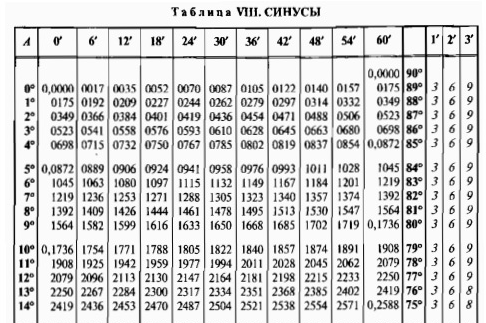Take for example the sinus table. Suppose one should determine what is equal to the value of the sine for an angle of 10 degrees and 30 minutes. We find in the left column the value of 10 degrees (11th line), and in the upper column - 30 minutes (6th column). At the intersection of 11 lines and the 6th column, we find the value of the function, 0.1822. The last three columns are designed to clarify the minutes of minutes. The fact is that only the values ​​of the minutes are presented in the top column of the values ​​6. To determine the sinus for other argument values, add or subtract the correction from the nearest function of the function presented in the table. For example, for an angle of 10 degrees and 32 minutes to the already found value of 0.1822, add correction from the second column, 6. So, the sinus of 10 degrees is 32 minutes will be 0.1822 + 0.0006 = 0.1828.

Since sinus and cosine, tangent and catangens for this angle are interrelated, the cosine values ​​can be determined on the sinus table, and the Tangent Table is the values ​​of the catangents. But the argument for cosine and for the catangent should be sought in the right column (fourth right) and at the bottom line.

Arguments of trigonometric functions in Bradys tables are set in degrees. To transfer degrees to radians, the value of the angle should be multiplied by 180 and divided by 3.1415926. By the way, the Tables of the Radian Angle Measure were also counted by V.M.Bradis and can be found in the brochure.

As you can see, Tables V.M.Bradis allow you to determine the four meaningful numbers of any function. Therefore, they are called "four-digit". Such accuracy of calculations is obviously enough for 90% of engineering calculations.

Currently, when the calculators are in hours, and in mobile phones, the calculations of the functions on the tables of the brady can be considered the "remnant of the past". But let's say honestly, the glorious past. I am very much seen at a distance. AND

Rockets then took off

...Published on the site

TopaverUseful links:
1. Who came up with Brady's tables?

## How to use the brady's table

The Bradys table is essentially not one table, but the collective name of the tables created by Mathematics V.M.Bradis in 1921, to calculate the values ​​of the trigonometric functions presented in degrees. Without them, to find the meaning of any function, there would have to make many complex computing. Now the brady's tables are used mainly to solve mathematical tasks in middle classes.

1

Why do you need Bradys tables?

In practice, Bradys tables are used when performing complex engineering calculations. Mathematics Vladimir Bradis, facilitated the task of calculating complex functions to many engineers and not only. Currently, all these functions can be calculated using a calculator, even on the usual phone.## 2The procedure for calculations on the Bradys table

Bradys tables There are several, they are called "four-digit tables", because the four important numbers are stored when calculating. There are tables for calculating the product of two-digit numbers, tables of squares and cubes, square roots, fractions, cosine, sinuses, tangents, catangents, logarithms and others. All these tables allow not to waste time on tedious calculations, but simply find a ready-made response to crossing rows and columns.## 3How to work on the brady's table?

Consider how to use the Bradys table in the calculations on the example of sinuses and cosine. In the top line, minutes are shown, in the extreme right column - degrees. Three extreme right columns are amendments for more accurate calculations.

• Danar: Find SIN 40 ° 30 '+ COS 32 ° 15'
• To find SIN 40 ° 30 'In the extreme left column, we find the value of 40 °, in the top line 30' and find their intersection. We get 0,6494.• To find the cosine value, the same table is used, but the degrees are in the fourth column from the edge to the right, and the minutes in the row from the bottom.
• We find the intersection of 32 ° and 12 ', because the table uses the minutes divided by 6. We get 0.8462.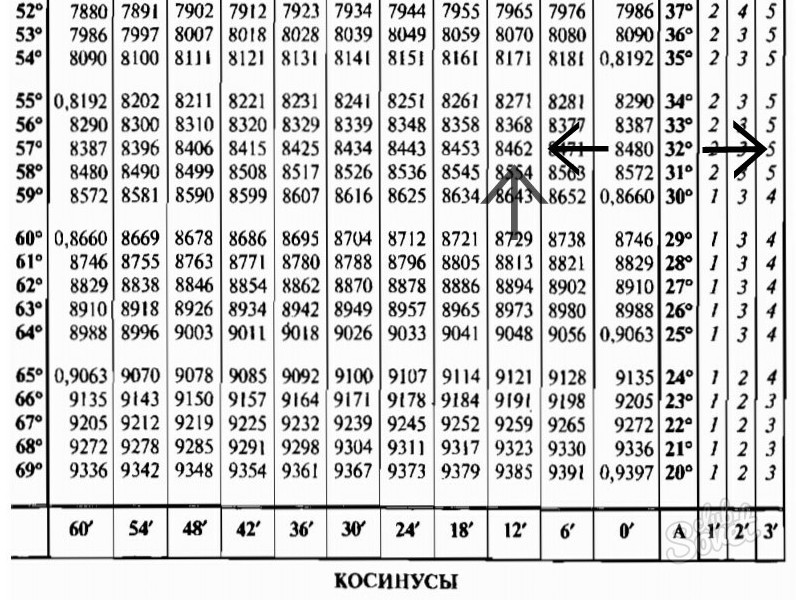• In the same line, we find the intersection with a column of the amendment to 3 'and add to 0.8462, because we need to find the value of 15'. It must be remembered that for cosine the amendment will have a negative sign. 0,8462 + (- 0.0005) = 0,8457
• Answer: SIN 40 ° 30 '+ COS 32 ° 15' = 0.6494 + 0.8457 = 1.4951.

So there is nothing complicated in the application of brady's tables. The main rules of which are attentiveness when finding values.

No matter how the computing technique was not improved, the definition of sinuses, cosine, tangents and catangers using Brady's tables It will always be relevant. Table Brady Created by an outstanding teacher-mathematician Vladimir Modestovich Bradys. In order for you to learn to use Brady's tables, which are presented below, we recommend first reading the instructions.

### Bradys Table - Instructions

1. Take the brand's table itself. If you do not have it in the printed form, then use our brady's tables. Open the appropriate chapter: tangents-catangents or cosine sines. For example, take sinus.
2.3. Make sure which angle you need to solve the problem. Brand's table can be applied without any problems, even when the angle is fractional, that is, its calculation occurs in degrees and minutes. If the magnitude of the angle is supplied in radians, convert its values ​​to degrees. It will be equal to the size of the size (considered in radians), multiplied by the ratio of 180 degrees to the value of π and is supplied by the general formula, namely: α Grad. = α. glad * 180 ° / π, with α Grad. The magnitude of the desired angle (is supplied in degrees), α glad - The value that is served in radians.
4. In the brady's table, you will be visible to some rows that will be horizontally, and vertically. Pay attention to the most extreme row located on the left. At the top of the left corner is the word sin, and under it there is a column from numbers with a degree name. This is a whole amount of degrees. Lay off the number that will directly correspond to the value of the whole degrees in the coal you already specified. For example, you can in the task angle equal to 27 ° 18 '. Please note that in the extreme left column there is a number 27. Then in the upper line, find the number 18. At the crossroads of the line and the column you can see the value you need.
5. Make an emphasis on the fact that degrees in the brady's table go among themselves in a row, and the minutes alternate after six. For example, 18 minutes in the table will be supplied, and 19 find you can no longer. To calculate the sinus of the desired angle, the amount of the minutes of which will not directly be more than 6, some amendments apply. They are located on the right side of the table. Consider the difference between the number of specified minutes in the right carbon and the closest corner, where the magnitude of the minute will be more than 6. If this difference is approximately 1, 2, 3 minutes, then you simply add the desired value to the final digit of the size of the sine of the smallest angle. If the difference wakes up close to 4 or 5, take the magnitude of the closest large angle and deduct from the final number of the first or second amendment.

### Bradys Table: Cosine sinuses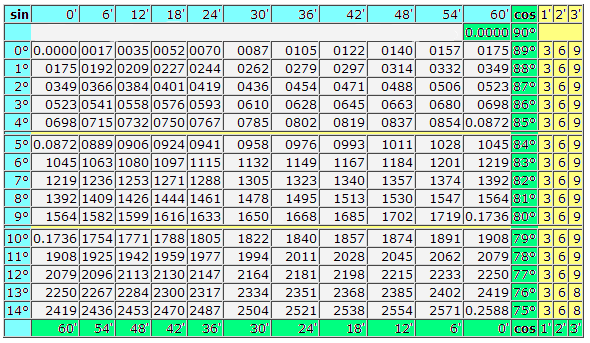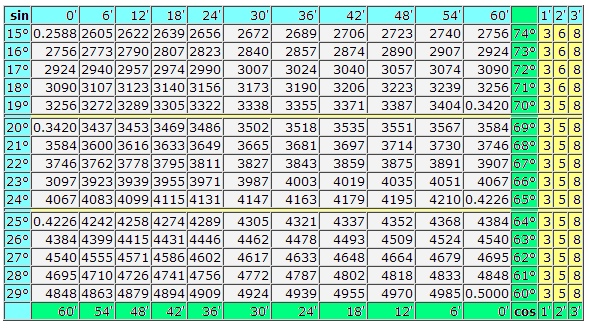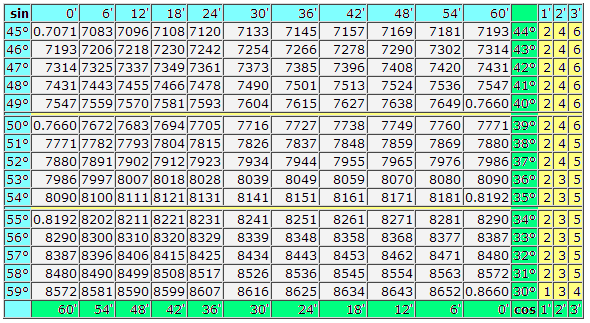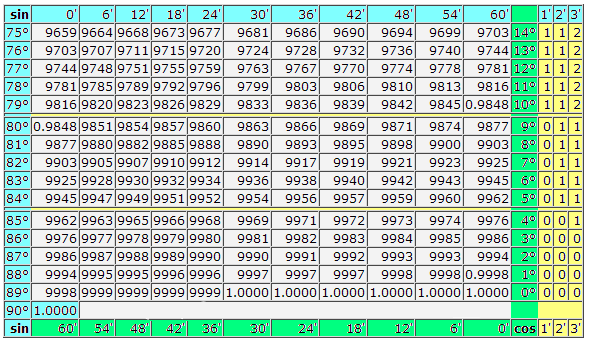### Brady's table: Tangents - Cotanges

TG and CTG large corners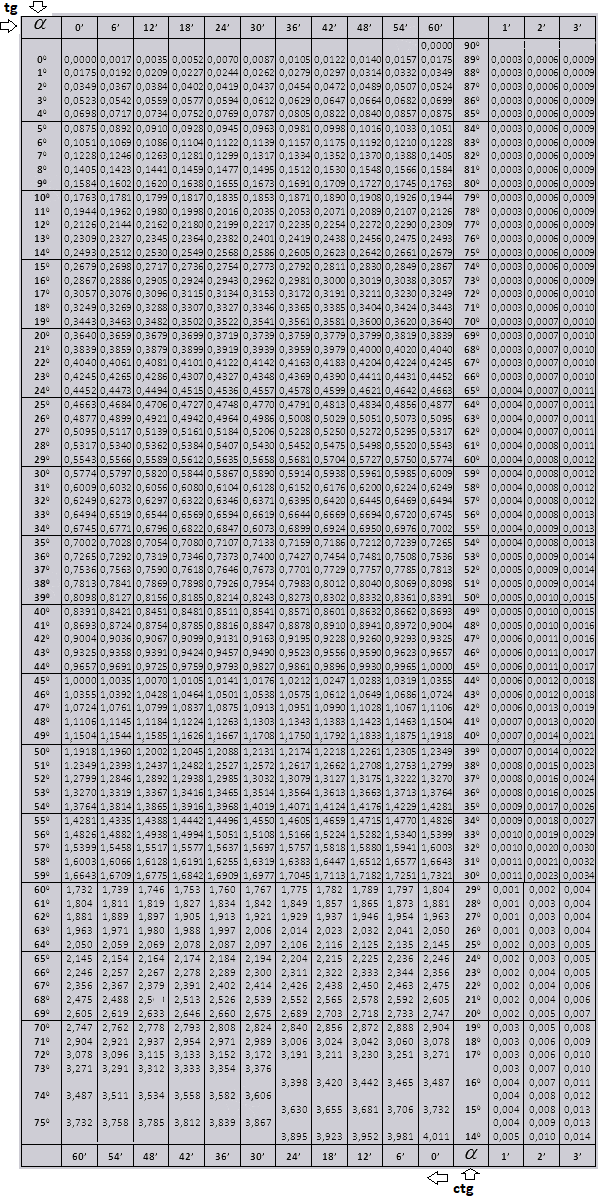TG and CTG Small CornersIf on use Brady's tables You have any questions, then write them in the comments. Thank you for using our service.

Muscovites may be interested in distance education in Moscow. Learn remotely - a gorgeous opportunity to become freer now.

If the material was useful, you can send donat Or share this material on social networks: